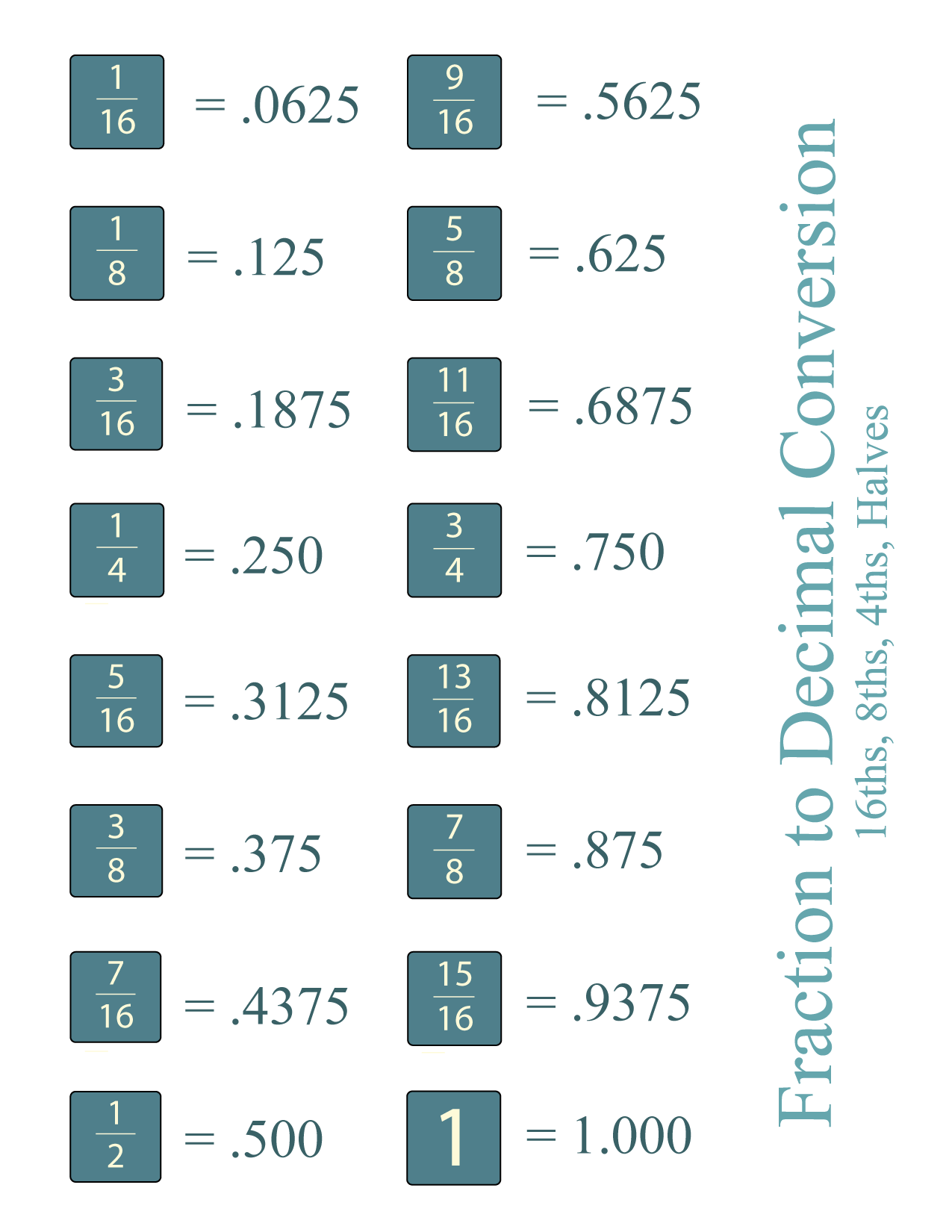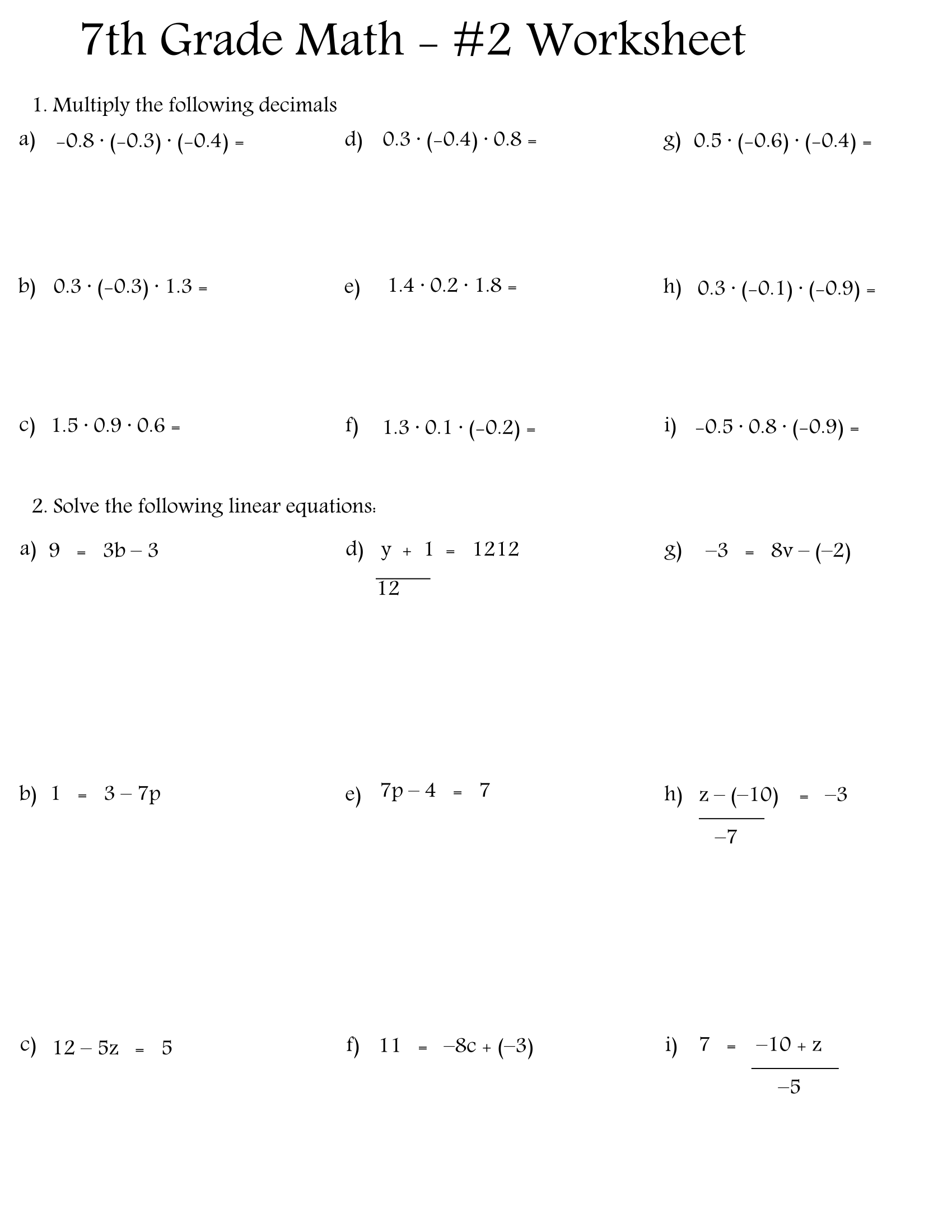## Free, printable 7th grade math worksheets (PDF)

Check out here the best free, printable 7th-grade math worksheets in PDF to prepare your kids for their next mathematical challenge. Are you worried that your kids won’t fully...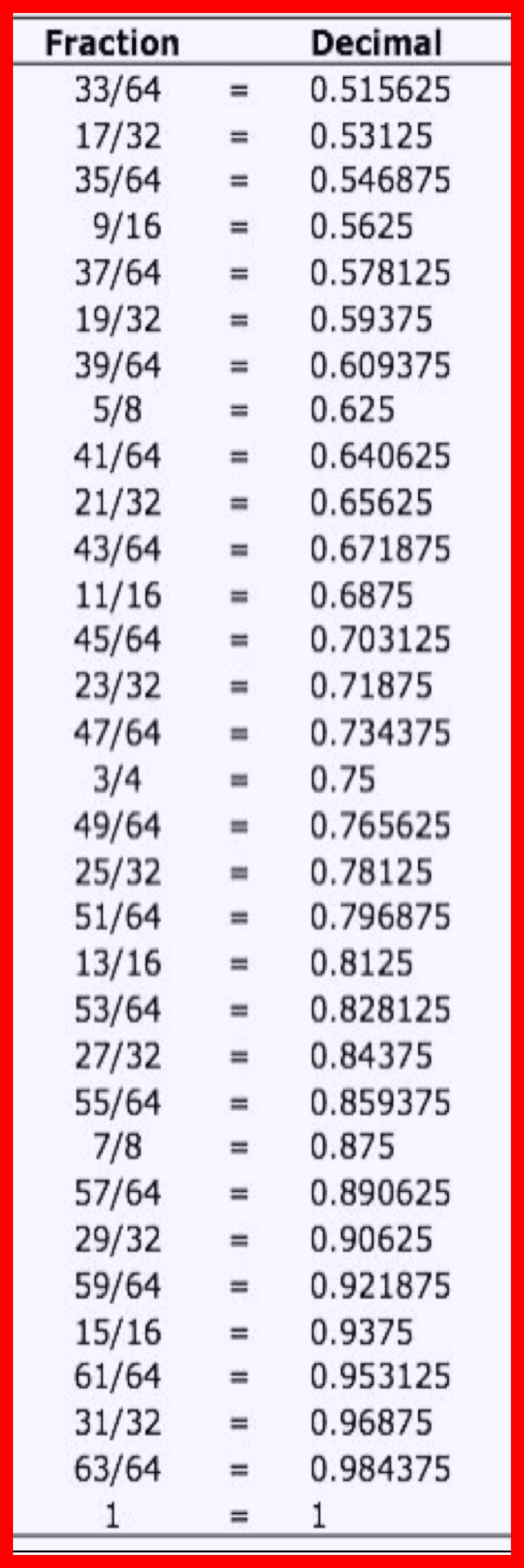## Printable inch fraction to decimal chart and cheatsheet

Get the best printable inch fraction to decimal chart here. Inch fractions and decimals are a bit complicated to master, especially if you don’t use them often. If you...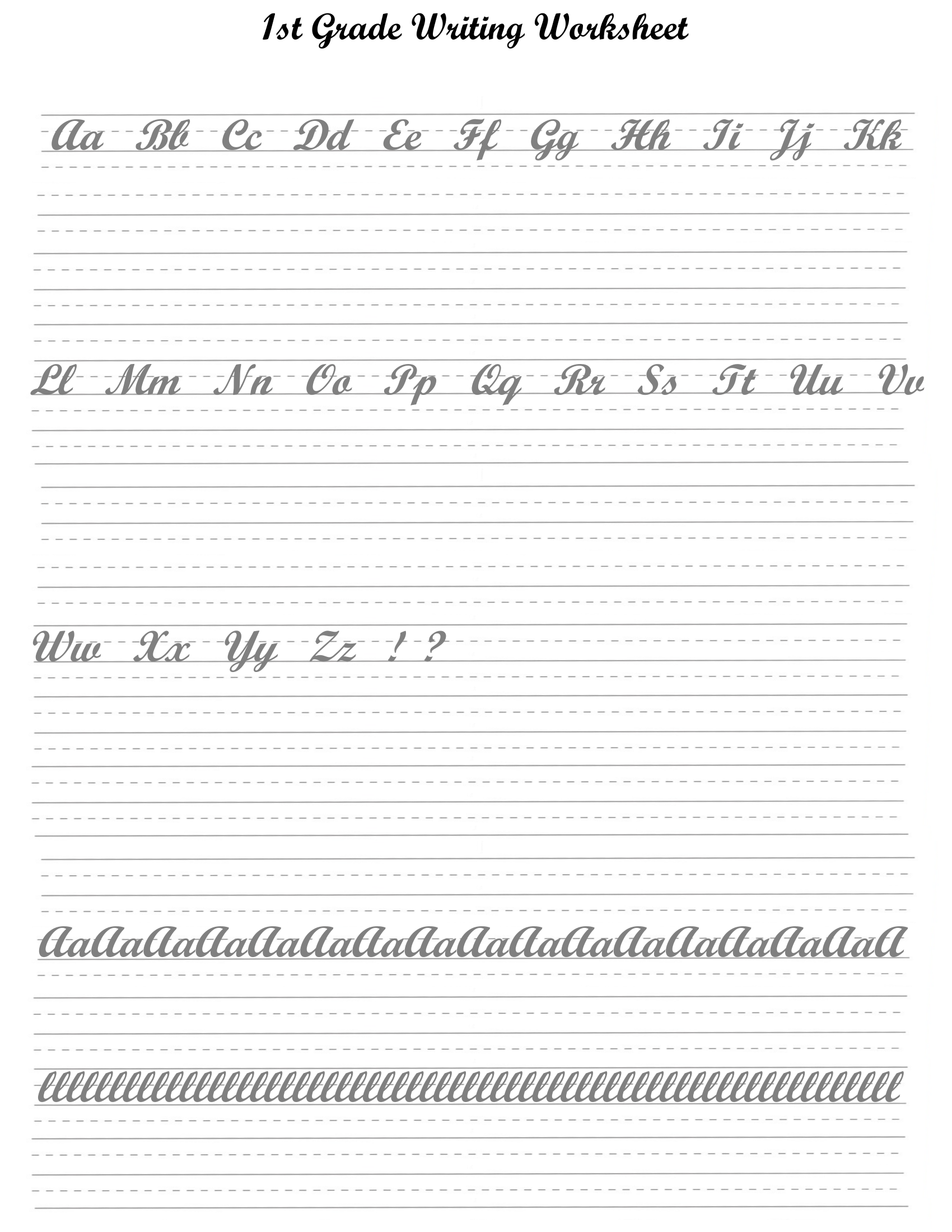## Free printable first grade worksheets for math, reading, and more (PDF)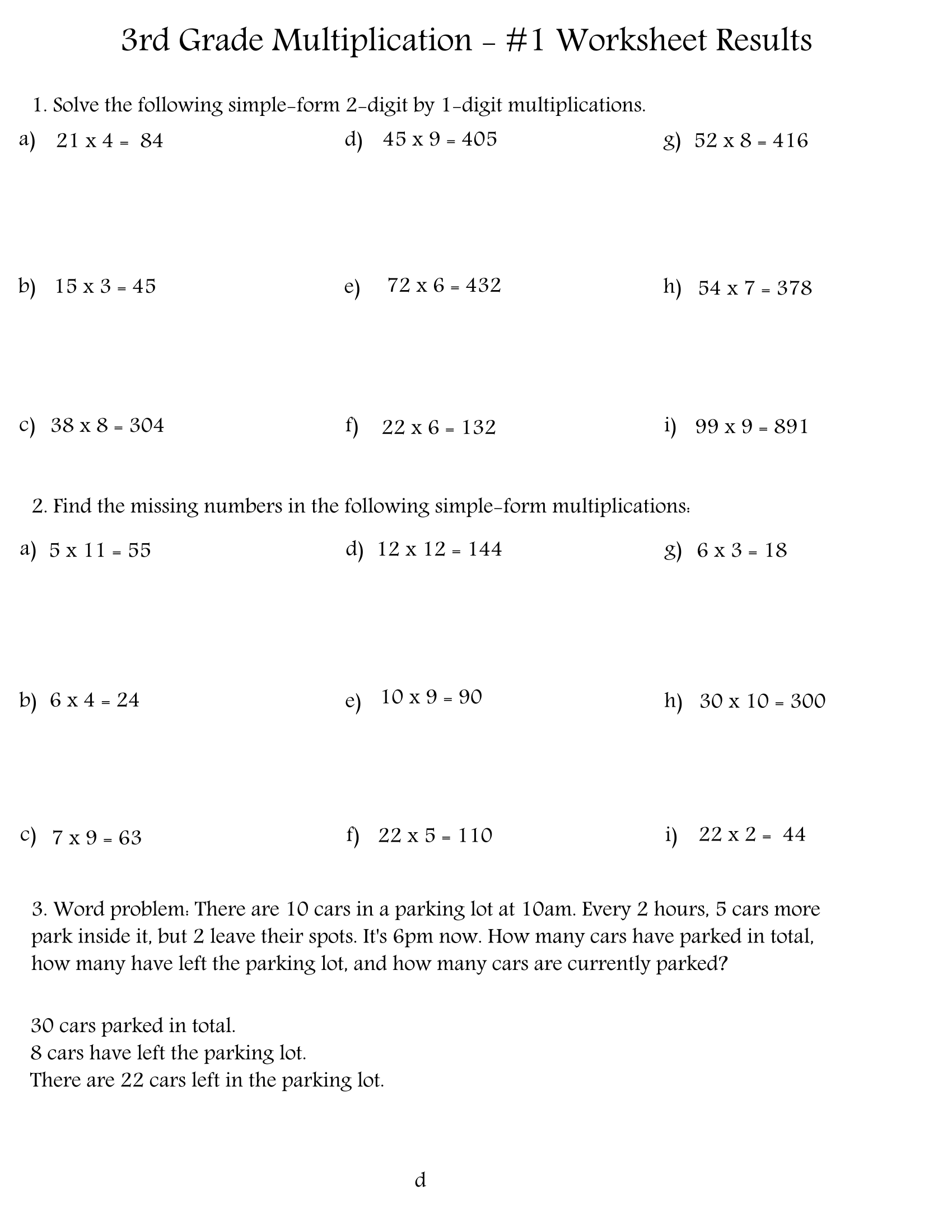## Printable 3rd grade multiplication worksheets and chart

3rd-grade multiplication worksheets are highly recommended tools for kids to master a new level of math difficulty that comes with this grade. Now, without school because of Covid-19, parents...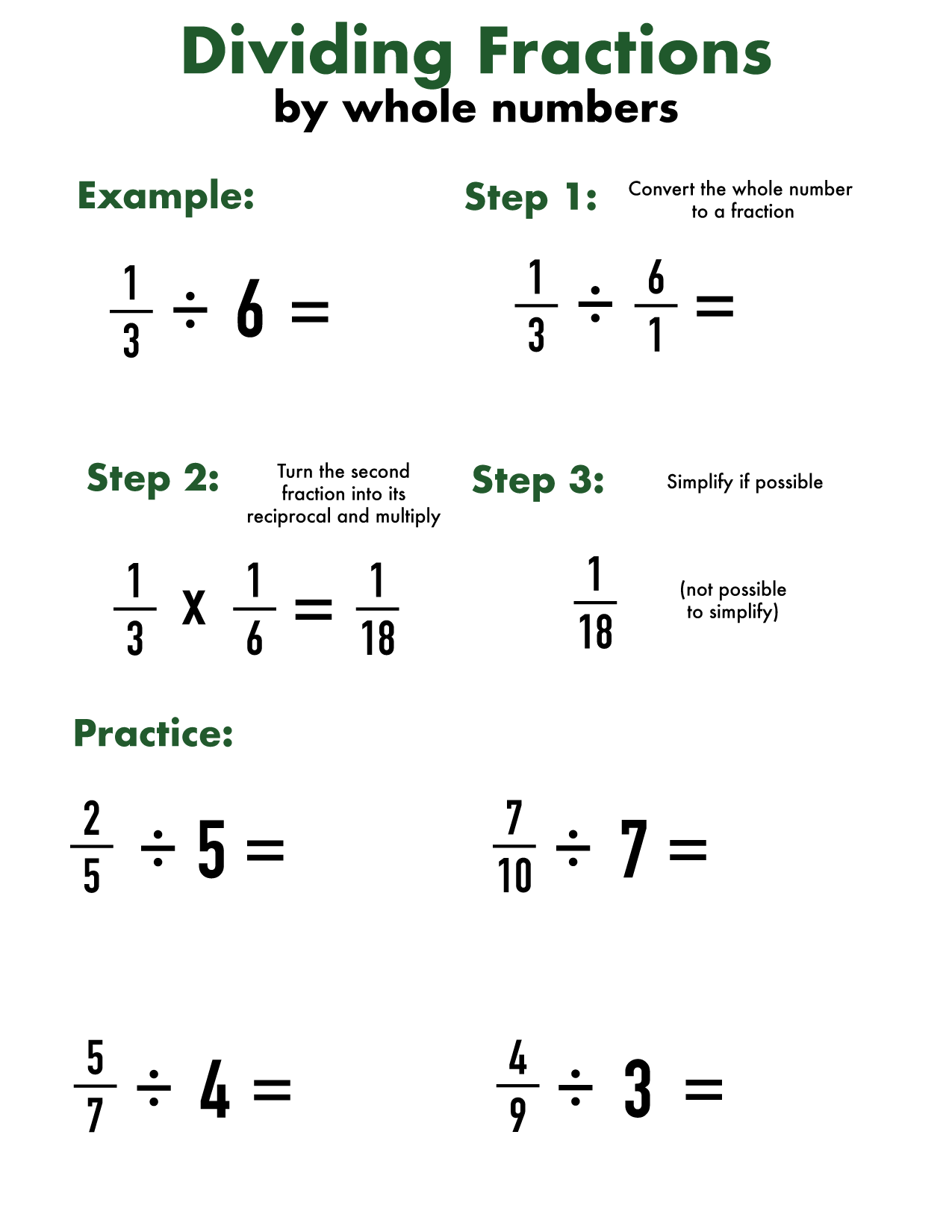## Printable dividing fractions by whole numbers worksheet (PDF)

Dividing fractions is the most difficult of functions involving fractions, but it’s not that hard once you get the hang of it! In this post, we will both give...

## Printable multiplication worksheets, charts and tables

Bring learning multiplication from an early math concept to real life with dozens of multiplication charts, multiplication tables, worksheets and games. Available for all ages, any grade and level,...## Printable multiplying fractions worksheets (by whole numbers) in PDF

Multiplying fractions is not that hard once you get used to it. In this post, we will get kids used to multiplying fractions by teaching them how it works...## Printable adding and subtracting fractions worksheets (with different or unlike denominators)

After students learn what fractions are, the next step is learning how to add and subtract them. Fractions with the same (or ‘like’) denominators are easier to add and...